# Python 樹表查找（二叉排序樹、平衡二叉樹）

## 什麼是樹表查詢？

`二叉排序樹``平衡二叉樹`

## 1. 二叉排序樹

`二叉樹`是樹結構中具有艷明特點的子類。

`二叉樹`要求樹的每一個結點（除葉結點）的子結點最多隻能有 `2` 個。在`二叉樹`的基礎上，繼續對其進行有序限制則變成`二叉排序樹`

### 1.1 構建一棵二叉排序樹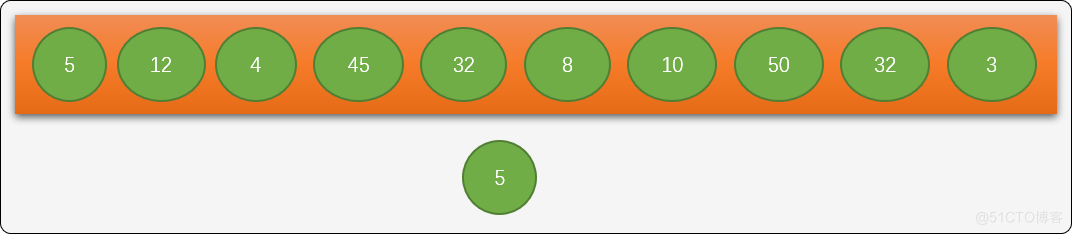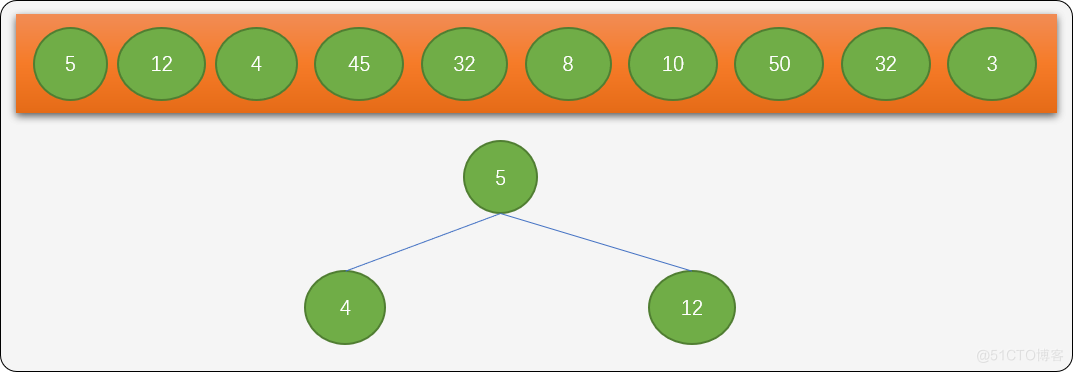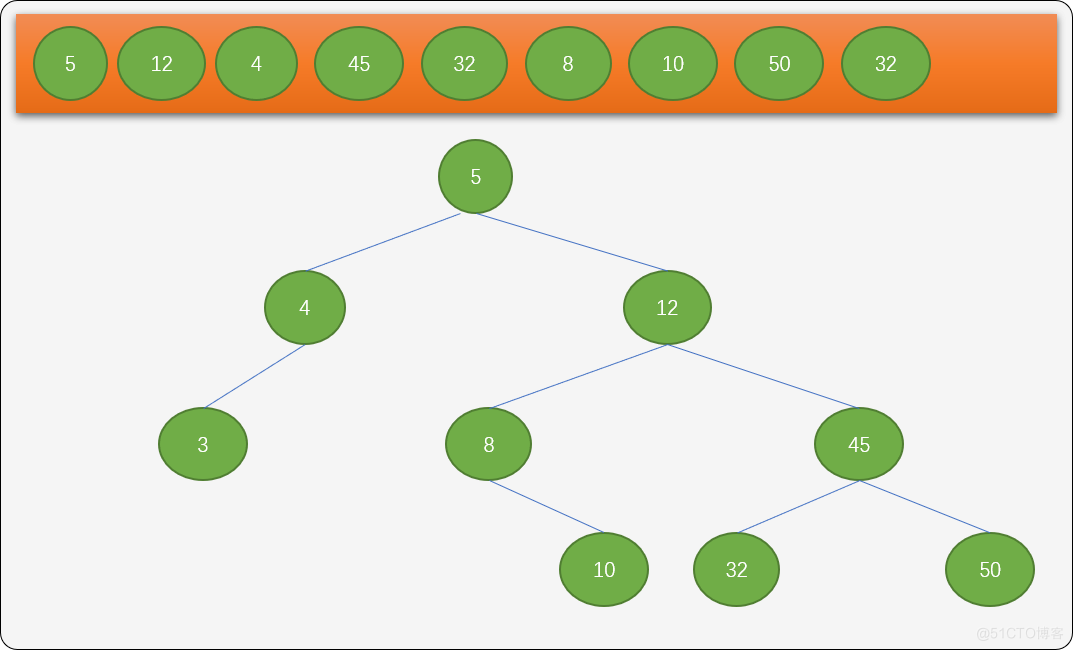### 1.2 二叉排序樹的數據結構

```'''

'''
class TreeNode():
def __init__(self, value):
# 結點上的值
self.value = value
# 左結點
self.l_child = None
# 右結點
self.r_child = None```

`value`：結點上附加的數據信息。 `l_child`：左子結點，初始值為 `None``r_child`：右子結點，初始值為 `None`

```'''

'''
class BinarySortTree:
# 初始化樹
def __init__(self, value=None):
pass

'''
在整棵樹上查詢是否存在給定的關鍵字
'''
def find(self, key):
pass

'''
使用遞歸進行查詢
'''
def find_dg(self, root, key):
pass

'''
插入新結點
'''
def insert(self, value):
pass

'''
中序遍歷
'''
def inorder_traversal(self):
pass
'''
刪除結點
'''
def delete(self, key):
pass

'''
檢查是不是空樹
'''
def is_empty(self):
return self.root == None```

### 1.3 實現二叉排序樹類中的方法：

`__init__` 初始化方法：

```    # 初始化樹
def __init__(self, value=None):
self.root = None
if value is not None:
root_node = TreeNode(value)
self.root = root_node```

```    '''
在整棵樹上查詢是否存在給定的關鍵字
key: 給定的關鍵字
'''
def find(self, key):
# 從根結點開始查找。
move_node = self.root
# 用來保存最後訪問過的結點
last_node = None
while move_node is not None:
# 保存當前結點
last_node = move_node
# 把關鍵字和當前結點相比較
if self.root.value == key:
# 出口一：成功查找
return move_node
elif move_node.value > key:
# 在左結點查找
move_node = move_node.l_child
else:
# 在右結點中查找
move_node = move_node.r_child
# 出口二：如果沒有查詢到，則返回最後訪問過的結點及None（None 表示沒查詢到）
return last_node, None```

```    '''
使用遞歸進行查詢
'''
def find_dg(self, root, key):
# 結點不存在
if root is None:
return None
# 相等
if root.value == key:
return root
if root.value > key:
return self.find_dg(root.l_child, key)
else:
return self.find_dg(root.r_child, key)```

`insert` 方法的實現：

```    '''
插入新結點
'''
def insert(self, value):
# 查詢是否存在此結點
res = self.find(value)
if type(res) != TreeNode:
# 沒找到，獲取查詢時最後訪問過的結點
last_node = res
# 創建新結點
new_node = TreeNode(value)
# 最後訪問的結點是根結點
if last_node is None:
self.root = new_node
if value > last_node.value:
last_node.r_child = new_node
else:
last_node.l_child = new_node``````if __name__ == "__main__":
nums = [5, 12, 4, 45, 32, 8, 10, 50, 32, 3]
tree = BinarySortTree(5)
for i in range(1, len(nums)):
tree.insert(nums[i])
print("測試根5 -> 左4 ->左3:")
tmp_node = tree.root
while tmp_node != None:
print(tmp_node.value, end=" ->")
tmp_node = tmp_node.l_child
print("\n測試根5 -> 右12 ->右45->右50：")
tmp_node = tree.root
while tmp_node != None:
print(tmp_node.value, end=" ->")
tmp_node = tmp_node.r_child
'''
輸出結果：
測試根5 -> 左4 ->左3:
5 ->4 ->3 ->
測試根5 -> 右12 ->右45->右50：
5 ->12 ->45 ->50 ->
''' ```

`二叉排序樹`進行中序遍歷，理論上輸出的數字應該是有序的。這裡寫一個中序遍歷，查看輸出的結點是不是有序的，從而驗證查詢和插入方法的正確性。

```    '''
中序遍歷
'''
def inorder_traversal(self, root):
if root is None:
return
self.inorder_traversal(root.l_child)
print(root.value,end="->")
self.inorder_traversal(root.r_child)```

```if __name__ == "__main__":
nums = [5, 12, 4, 45, 32, 8, 10, 50, 32, 3]
tree = BinarySortTree(5)
# res = tree.find(51)
for i in range(1, len(nums)):
tree.insert(nums[i])
tree.inorder_traversal(tree.root)
'''
輸出結果
3->4->5->8->10->12->32->45->50->
'''```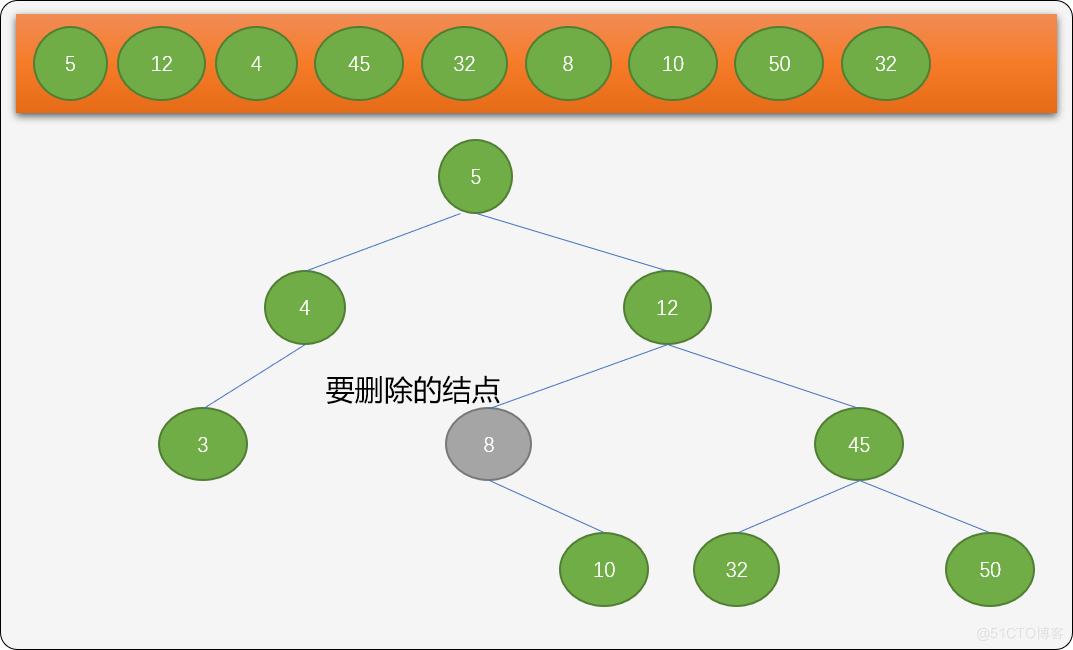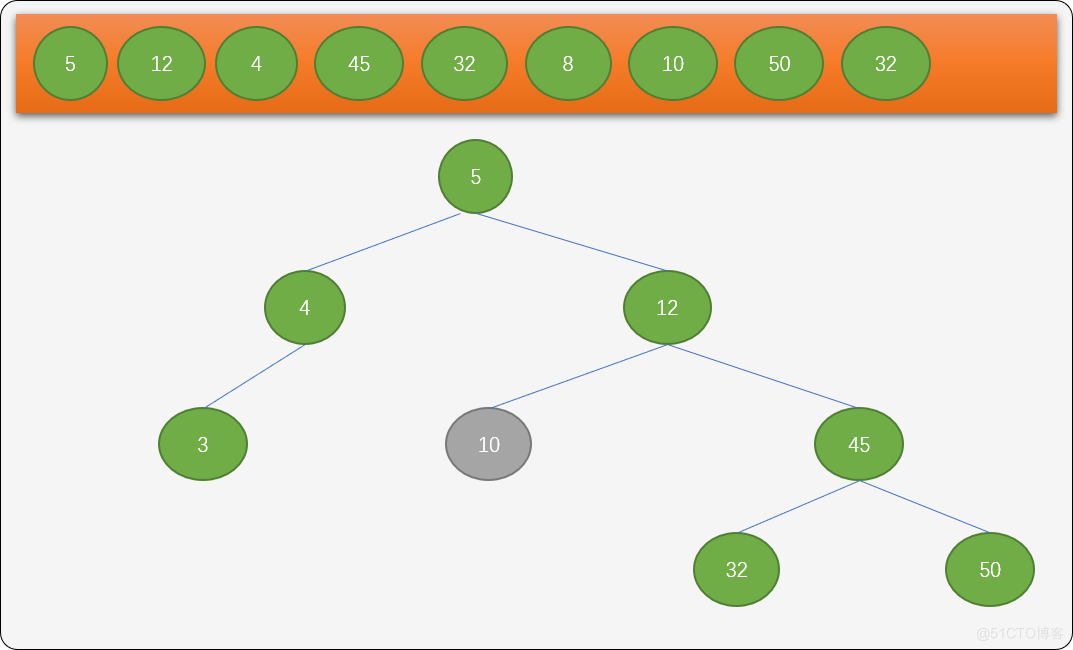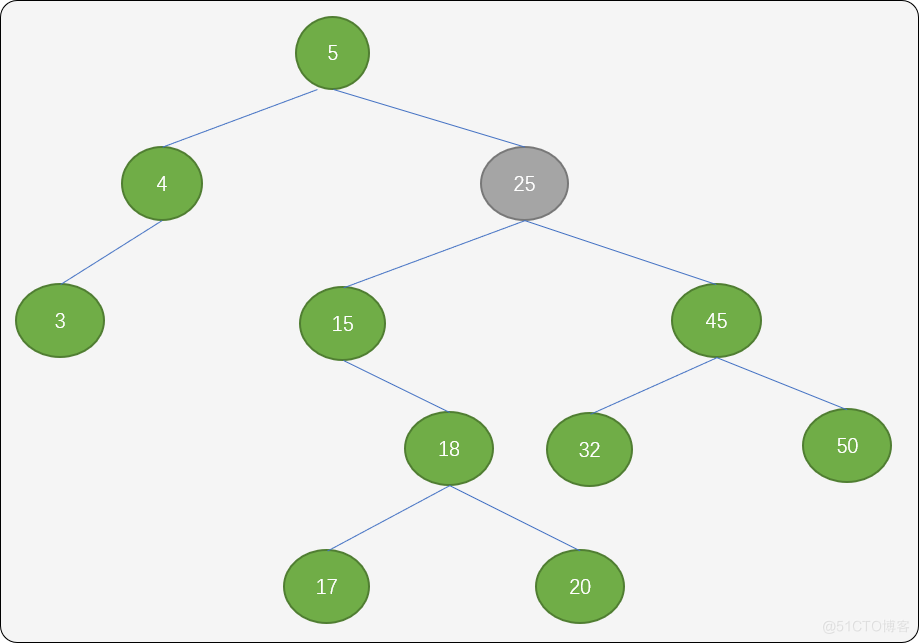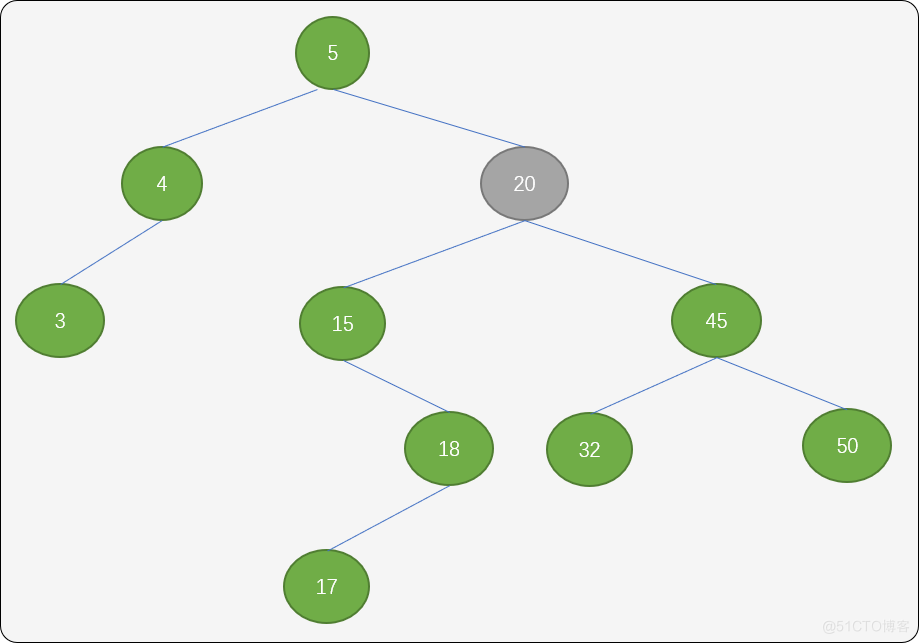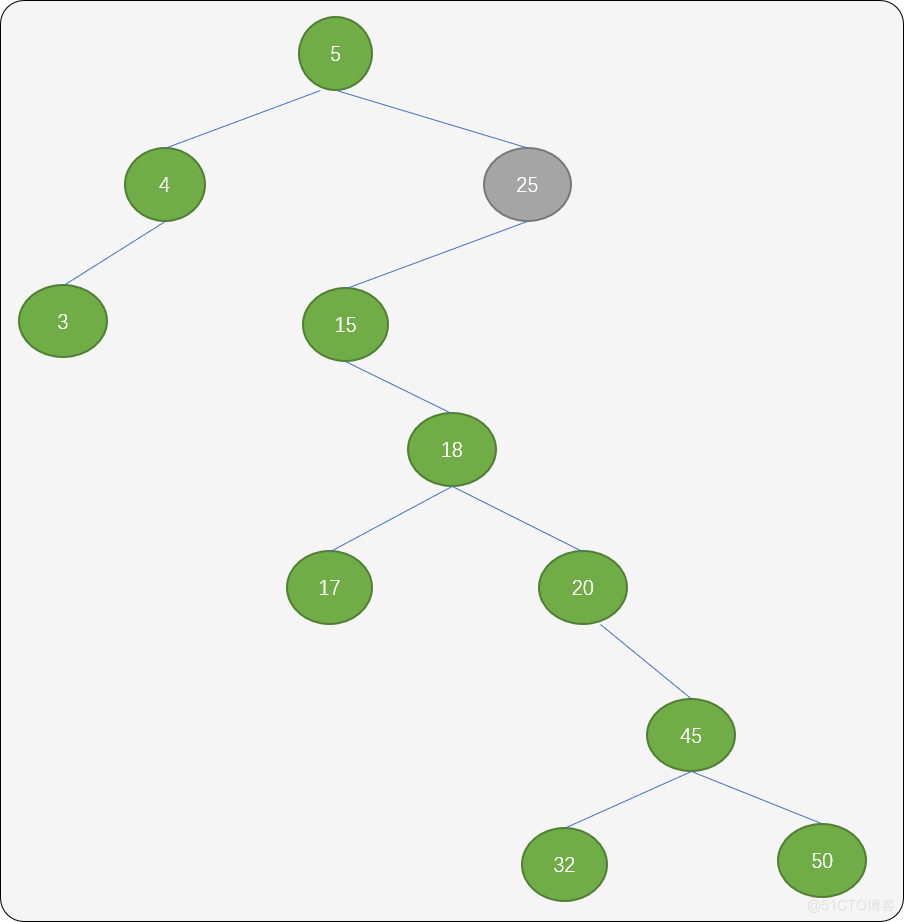``` 	'''
刪除結點
key 為要要刪除的結點
'''
def delete(self, key):
# 從根結點開始查找，move_node 為搜索指針
move_node = self.root
# 要刪除的結點的父結點,因為根結點沒有父結點，初始值為 None
parent_node = None
# 結點存在且沒有匹配上要找的關鍵字
while move_node is not None and move_node.value != key:
# 保證當前結點
parent_node = move_node
if move_node.value > key:
# 在左子樹中繼續查找
move_node = move_node.l_child
else:
# 在右子樹中繼續查找
move_node = move_node.r_child
# 如果不存在
if move_node is None:
return -1
# 檢查要刪除的結點是否存在左子結點
if move_node.l_child is None:
if parent_node is None:
# 如果要刪除的結點是根結點
self.root = move_node.r_child
elif parent_node.l_child == move_node:
# 刪除結點的右結點作為父結點的左結點
parent_node.l_child = move_node.r_child
elif parent_node.r_child == move_node:
parent_node.r_child = move_node.r_child
return 1
else:
# 如果刪除的結點存在左子結點,則在左子樹中查找最大值
s = move_node.l_child
q = move_node
while s.r_child is not None:
q = s
s = s.r_child
if q == move_node:
move_node.l_child = s.l_child
else:
q.r_child = s.l_child
move_node.value = s.value
q.r_child = None
return 1```

```if __name__ == "__main__":
nums = [5, 12, 4, 45, 32, 8, 10, 50, 32, 3]
tree = BinarySortTree(5)
# res = tree.find(51)
for i in range(1, len(nums)):
tree.insert(nums[i])
tree.delete(12)
tree.inorder_traversal(tree.root)
'''
輸出結果
3->4->5->8->10->32->45->50->
'''```

## 3. 平衡二叉排序樹

`二叉排序樹`中進行查找時，其`時間復雜度`理論上接近`二分算法`的時間復雜度，其查找時間與樹的深度有關。但是，這裡有一個問題，前面討論過，如果數列中的數字順序不一樣時，所構建出來的二叉排序樹的深度會有差異性，對最後評估時間性能也會有影響。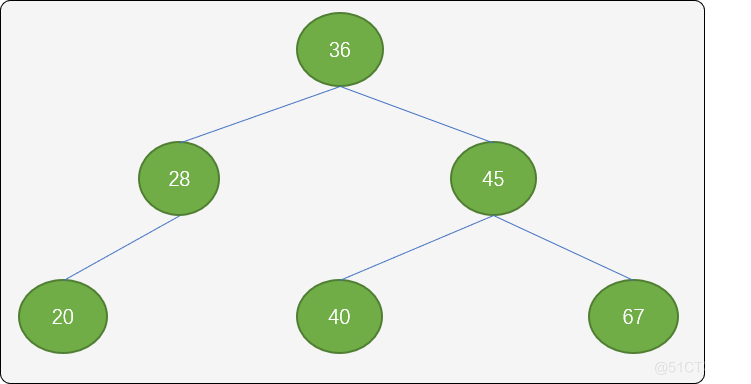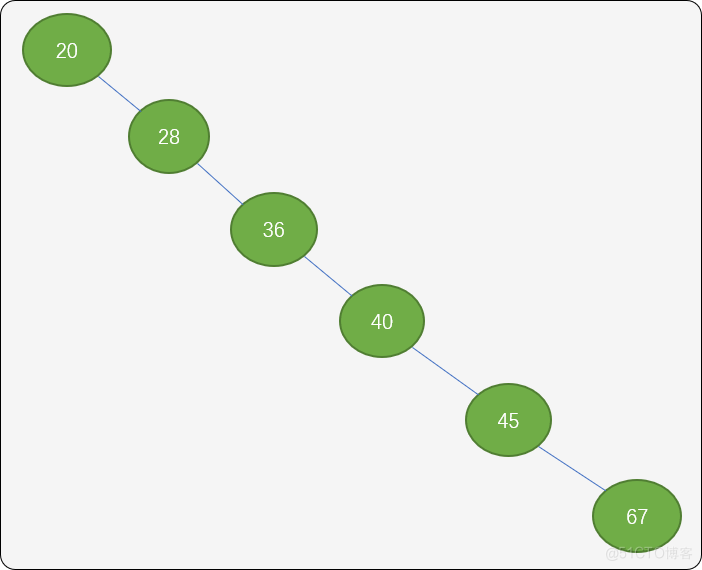`0` ：左、右子樹深度一樣。 `1`：左子樹深度大於右子樹。 `-1`：左子樹深度小於右子樹。### 3.1 二叉平衡排序樹的數據結構

```'''

'''
class TreeNode:
def __init__(self,value):
self.value=value
self.l_child=None
self.r_child=None
self.balance=0```

`value`：結點上附加的值。 `l_child`：左子結點。 `r_child`：右子結點。 `balance`：平衡因子，默認平衡因子為 `0`

```'''

'''
class Tree:
def __init__(self, value):
self.root = None

'''
LL型調整
'''
def ll_rotate(self, node):
pass

'''
RR 型調整
'''
def rr_rotate(self, node):
pass

'''
LR型調整
'''
def lr_rotate(self, node):
pass

'''
RL型調整
'''
def rl_rotate(self, node):
pass

'''
插入新結點
'''
def insert(self, value):
pass

'''
中序遍歷
'''
def inorder_traversal(self, root):
pass

def is_empty(self):
pass```

`LL型調整（順時針）`：左邊不平衡時，向右邊旋轉。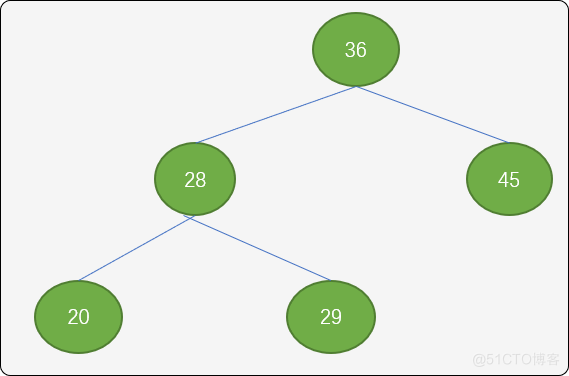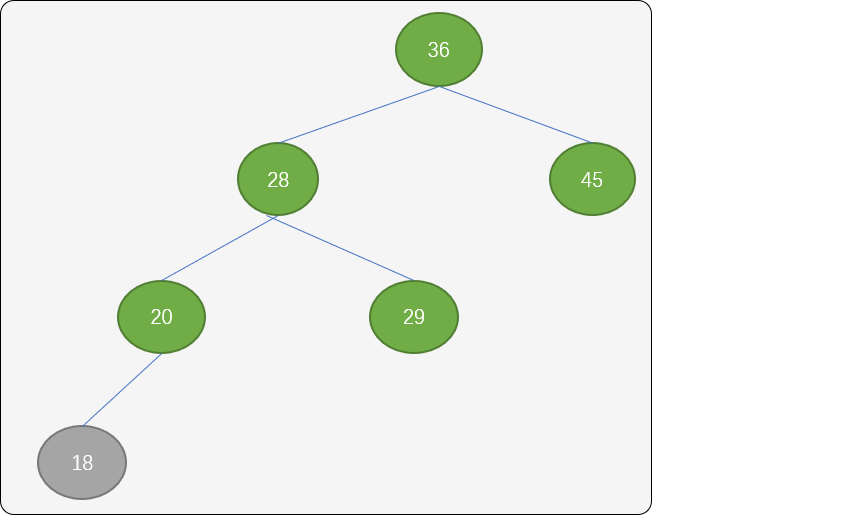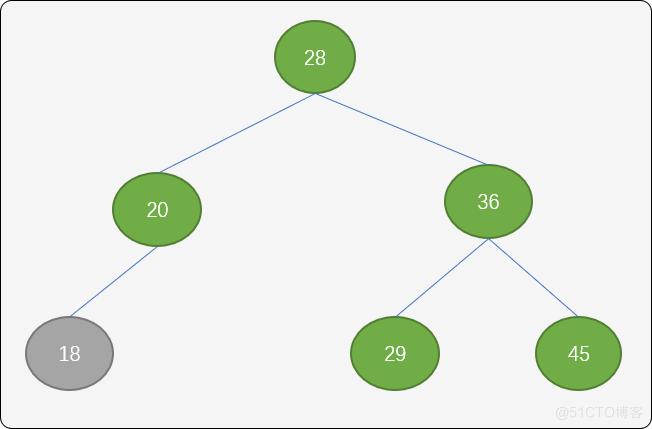`LL` 旋轉算法具體實現：

```    '''
LL型調整
順時針對調整
'''
def ll_rotate(self, p_root):
# 原父結點的左子結點成為新父結點
new_p_root = p_root.l_child
# 新父結點的右子結點成為原父結點的左子結點
p_root.l_child = new_p_root.r_child
# 原父結點成為新父結點的右子結點
new_p_root.r_child = p_root
# 重置平衡因子
p_root.balance = 0
new_p_root.balance = 0
return new_p_root```

`RR 型調整(逆時針旋轉)``RR`旋轉和 `LL`旋轉的算法差不多，隻是當右邊不平衡時，向左邊旋轉。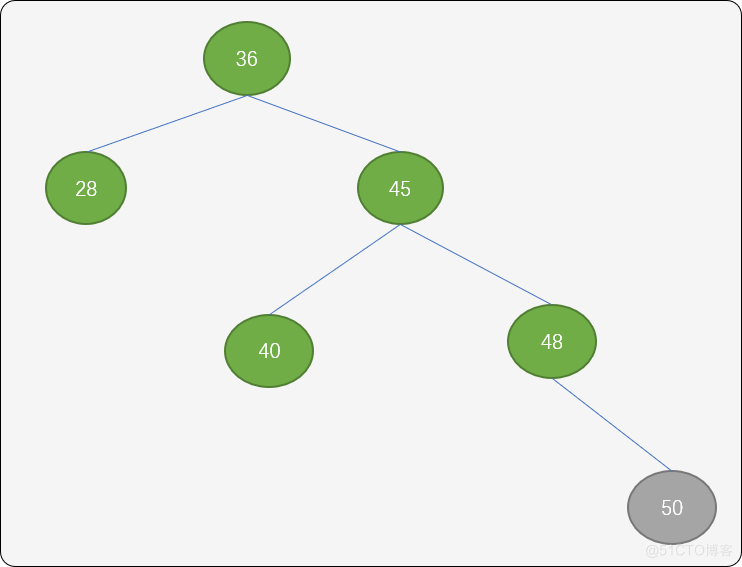`RR` 旋轉算法具體實現：

```    '''
RR 型調整
'''
def rr_rotate(self, node):
# 右子結點
new_p_node = p_node.r_child
p_node.r_child = new_p_node.l_child
new_p_node.l_child = p_node
# 重置平衡因子
p_node.balance = 0
new_p_node.balance = 0
return new_p_node```

**`LR型調整（先逆後順）`：**如下圖當插入結點 `28` 後，結點 `36` 的平衡因子變成 `2`，則可以使用 `LR` 旋轉算法。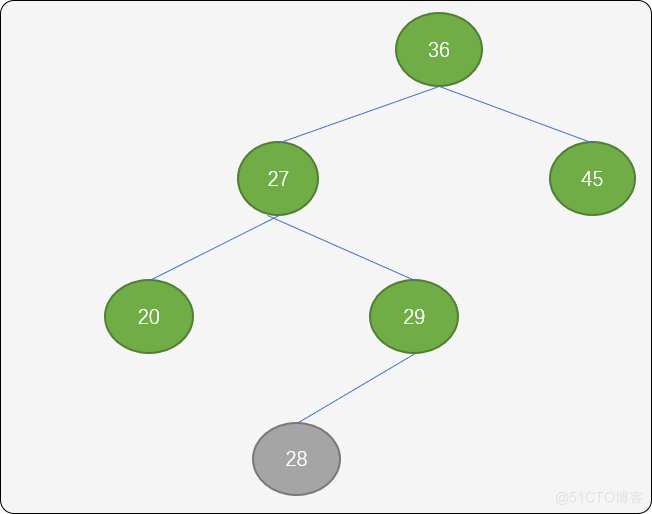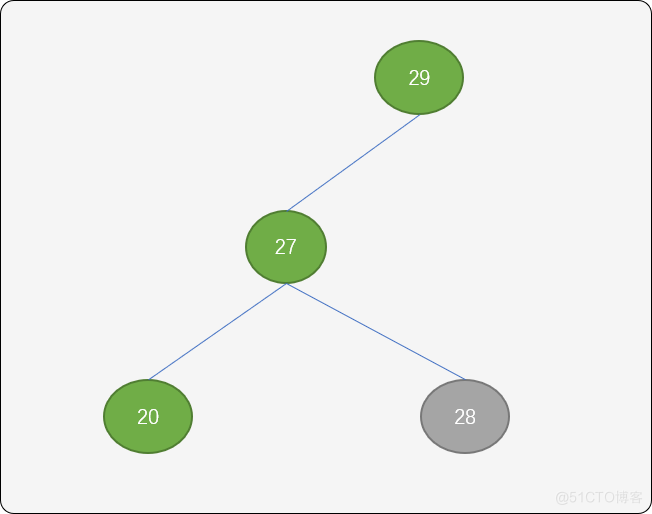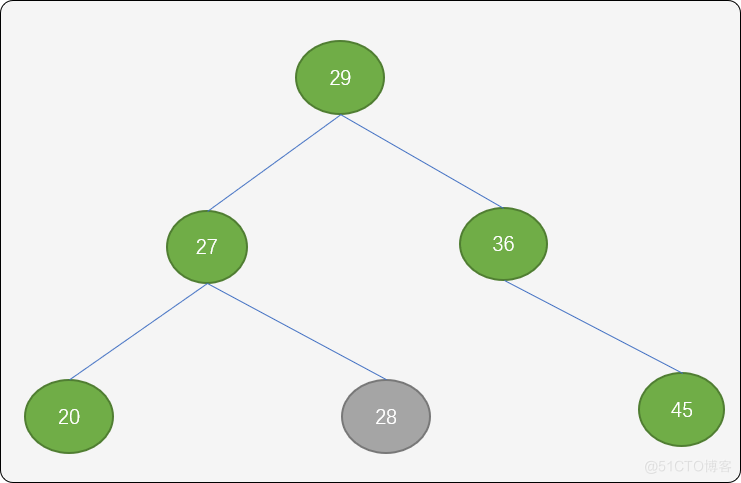`LR` 旋轉算法實現：

```    '''
LR型調整
'''
def lr_rotate(self, p_node):
# 左子結點
b = p_node.l_child
new_p_node = b.r_child
p_node.l_child = new_p_node.r_child
b.r_child = new_p_node.l_child
new_p_node.l_child = b
new_p_node.r_child = p_node
if new_p_node.balance == 1:
p_node.balance = -1
b.balance = 0
elif new_p_node.balance == -1:
p_node.balance = 0
b.balance = 1
else:
p_node.balance = 0
b.balance = 0
new_p_node.balance = 0
return new_p_node```

`RL`型調整： 如下圖插入結點`39` 後，整棵樹的平衡打破，這時可以使用 `RL` 旋轉算法進行調整。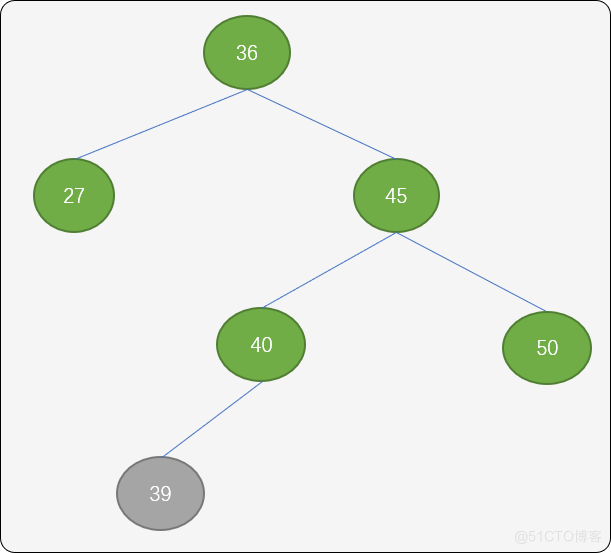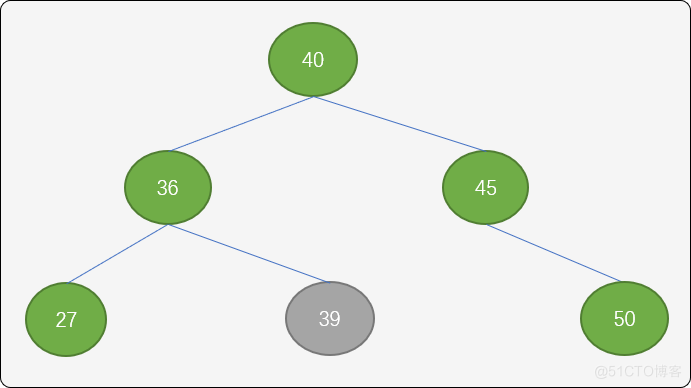`RL` 算法具體實現：

```    '''
RL型調整
'''
def rl_rotate(self, p_node):
b = p_node.r_child
new_p_node = b.l_child
p_node.r_child = new_p_node.l_child
b.l_child = new_p_node.r_child
new_p_node.l_child = p_node
new_p_node.r_child = b
if new_p_node.balance == 1:
p_node.balance = 0
b.balance = -1
elif new_p_node.balance == -1:
p_node.balance = 1
b.balance = 0
else:
p_node.balance = 0
b.balance = 0
new_p_node.balance = 0
return new_p_node```

```    '''
插入新結點
'''
def insert(self, val):
# 新的結點
new_node = TreeNode(val)
if self.root is None:
# 空樹
self.root = new_node
return
# 記錄離 s 最近的平衡因子不為 0 的結點。
min_b = self.root
# f 指向 a 的父結點
f_node = None
move_node = self.root
f_move_node = None
while move_node is not None:
if move_node.value == new_node.value:
# 結點已經存在
return
if move_node.balance != 0:
# 尋找最小不平衡子樹
min_b = move_node
f_node = f_move_node
f_move_node = move_node
if new_node.value < move_node.value:
move_node = move_node.l_child
else:
move_node = move_node.r_child

if new_node.value < f_move_node.value:
f_move_node.l_child = new_node
else:
f_move_node.r_child = new_node
move_node = min_b
# 修改相關結點的平衡因子
while move_node != new_node:
if new_node.value < move_node.value:
move_node.balance += 1
move_node = move_node.l_child
else:
move_node.balance -= 1
move_node = move_node.r_child

if min_b.balance > -2 and min_b.balance < 2:
# 插入結點後沒有破壞平衡性
return

if min_b.balance == 2:
b = min_b.l_child
if b.balance == 1:
move_node = self.ll_rotate(min_b)
else:
move_node = self.lr_rotate(min_b)
else:
b = min_b.r_child
if b.balance == 1:
move_node = self.rl_rotate(min_b)
else:
move_node = self.rr_rotate(min_b)
if f_node is None:
self.root = move_node
elif f_node.l_child == min_b:
f_node.l_child = move_node
else:
f_node.r_child = move_node```

```    '''
中序遍歷
'''
def inorder_traversal(self, root):
if root is None:
return
self.inorder_traversal(root.l_child)
print(root.value, end="->")
self.inorder_traversal(root.r_child)```

```if __name__ == "__main__":
nums = [3, 12, 8, 10, 9, 1, 7]
tree = Tree(3)
for i in range(1, len(nums)):
tree.inster(nums[i])
# 中序遍歷
tree.inorder_traversal(tree.root)
'''
輸出結果
1->3->7->8->9->10->12->
'''```